Volume 377, Issues 45–48, 17 December 2013, Pages 3247–3253

# Tachyonic Cherenkov emission from Jupiterʼs radio electrons

• Department of Physics, Hiroshima University, 1-3-1 Kagami-yama, Higashi-Hiroshima 739-8526, Japan

## Highlights

Tachyonic radio emission from uniformly moving electrons in a dispersive spacetime.

Transversal and longitudinal flux densities due to superluminal Cherenkov effect.

Mildly relativistic thermal electron populations in Jupiterʼs radiation belts.

Spectral fit of ground-based and in-situ measurements of the Jovian radio flux.

Jupiterʼs low flux density at 13.8 GHz (Cassini fly-by) due to thermal spectral cutoff.

## Abstract

Tachyonic Cherenkov radiation from inertial relativistic electrons in the Jovian radiation belts is studied. The tachyonic modes are coupled to a frequency-dependent permeability tensor and admit a negative mass-square, rendering them superluminal and dispersive. The superluminal radiation field can be cast into Maxwellian form, using 3D field strengths and inductions, and the spectral densities of tachyonic Cherenkov radiation are derived. The negative mass-square gives rise to a longitudinal flux component. A spectral fit to Jupiterʼs radio spectrum, inferred from ground-based observations and the Cassini 2001 fly-by, is performed with tachyonic Cherenkov flux densities averaged over a thermal electron population.

## Keywords

• Superluminal radiation modes with negative mass-square;
• Transversal and longitudinal tachyons;
• Tachyonic Maxwell–Proca fields in a permeable spacetime;
• Thermal electron plasma in Jupiterʼs radiation belts;
• Cassiniʼs Jupiter fly-by and Jovian radio spectrum

## 1. Introduction

During the Cassini Jupiter fly-by in January 2001, an in situ measurement of the Jovian radio emission was performed, resulting in an unexpectedly low flux density of 0.44±0.15 Jy at 13.8 GHz, as compared to ground-based observations at 8.6 GHz, which produced an averaged flux of 2.3±0.6 Jy. This sudden decline is hard to explain with magnetospheric ultra-relativistic synchrotron radiation models. Here, we investigate the presently available radio spectrum ranging from 74 MHz up to the Cassini flux at 13.8 GHz. We perform a spectral fit to the Jovian radio flux with a tachyonic Cherenkov density , ,  and  produced by mildly relativistic thermal electrons in the radiation belts. The superluminal radiation field (Proca field) satisfies Maxwellʼs equations with negative mass-square . On that basis, we derive the tachyonic Cherenkov flux generated by inertial charges propagating in a dispersive spacetime.

In Section 2, we discuss Proca fields with negative mass-square and frequency-dependent permeabilities. We outline the tachyonic Maxwell equations in terms of 3D field strengths and inductions and develop the 4D Lagrange formalism using dispersive permeability tensors. We obtain field equations which have a manifestly covariant appearance, even though the permeability tensors are a manifestation of the absolute spacetime required for causal superluminal signal transfer ,  and .

In Section 3, we analyze asymptotic tachyonic radiation fields generated by a classical subluminal current density, and decompose them into transversal and longitudinal field components. In Section 4, we derive the tachyonic Cherenkov flux densities of a relativistic charge in uniform motion. A longitudinal radiation component emerges whose intensity scales with the negative mass-square of the radiation field. As in the case of electromagnetic Cherenkov radiation, the mass of the radiating particle does not enter in the tachyonic Cherenkov densities. This gives credence to the view , that Cherenkov radiation is not so much radiation by a charge passing through a medium, but rather radiation by the medium itself, excited by the field of the inertial charge.

In Section 5, we average the differential tachyonic Cherenkov flux over relativistic electron distributions (thermal Maxwell–Boltzmann and nonthermal power-law distributions). In Section 6.1, we discuss spectral fitting with Cherenkov flux densities, adapted to Jupiterʼs radio band. In Section 6.2, a spectral fit to the Jovian radio emission is performed. The low flux density at 13.8 GHz measured by the Cassini spacecraft can well be explained by tachyonic Cherenkov emission from thermal electrons in Jupiterʼs radiation belts, the Cassini data point being located in the exponentially decaying spectral tail. In Section 7, we present our conclusions.

## 2. Tachyonic Proca–Maxwell fields: manifestly covariant field equations in a permeable spacetime

We start with some conventions regarding the Fourier time transform. The vector potential Aμ=(A0,A) transforms as , and the same holds for the field strengths, inductions and the current. Since Aμ is real, . The homogeneous Maxwell equations in space–frequency representation read , . The field strengths and are related to the vector potential by , . The constitutive equations defining the inductions and and the inductive potential read  and 

equation2.1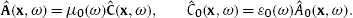The permeabilities ε(ω), μ(ω), μ0(ω) and ε0(ω) are independent of the space variable, real and symmetric ε(ω)=ε(−ω). The inhomogeneous field equations coupled to a current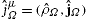(which will be defined in (2.5)) read
equation2.2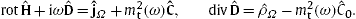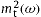is the negative tachyonic mass-square (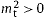in our sign convention), which can be frequency-dependent like the permeabilities,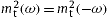. We take the divergence of the first equation in (2.2) and substitute the second, to obtain the Lorentz condition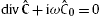, subject to current conservation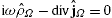. The corresponding Fourier representation of the Poynting flux vector  is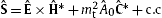.

To write the Maxwell equations manifestly covariantly in Fourier space, we start with the field tensor Fμν(x,t)=Aν,μAμ,ν. We use the convention that time differentiation in Fourier space means to multiply with a factor −iω, e.g.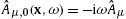. For conjugated fields,. Thus,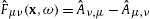and, which actually means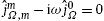. The 3D field strengths areand, and inversely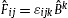, where εkij is the Levi-Civita 3-tensor. The manifestly covariant homogeneous field equations read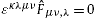, where εκλμν is the totally antisymmetric 4-tensor.

The permeabilities (ε0(ω),μ0(ω)) and (ε(ω),μ(ω)) in (2.1) define isotropic real and symmetric permeability tensors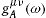and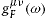,

equation2.3with zero flanks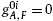and. Greek indices are raised and lowered with the Minkowski metric ημν=diag(−1,1,1,1). We may then write the inductions (2.1) manifestly covariantly as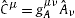,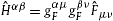, so that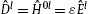and, and inversely. Also,and.

The action functional is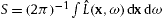, defined by the Lagrangian

equation2.4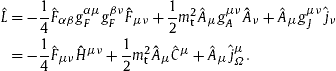The coupling of the wave modes to an external currentis effected by a permeability tensor,

equation2.5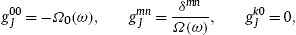with the same properties as the tensorsin (2.3). In the field equations (2.2), we use the ‘dressed’ current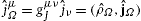. Euler variation of the action gives the manifestly covariant field equations Hˆμν,νm2tCˆμ=jˆμΩ in space–frequency (x,ω) representation, equivalent to (2.2). (Hˆm0,0(x,ω)=iωHˆm0, as defined above.) If the current jˆμΩ is conserved, jˆμΩ,μ=0, we find the Lorentz condition Cˆμ,μ=0. The permeability tensor (2.5) amounts to a frequency-dependent coupling constant in Lagrangian (2.4) if Ω0(ω)=1/Ω(ω), which we assume in the following, jˆμΩ=jˆμ/Ω(ω). Thus, if the external current is conserved, jˆm,miωjˆ0=0, this also holds for the dressed current jˆμΩ. In fact, Ω(ω) can be scaled into the permeabilities (2.1), εΩε, μμ/Ω, and analogously for (ε0,μ0), cf. (2.2).

## 3. Asymptotic radiation fields: time-averaged transversal and longitudinal energy flux

The tachyonic Maxwell equations (stated in (2.2) and after (2.5), with permeability tensors in (2.3) and (2.5)) are solved by the transversal and longitudinal asymptotic vector potentials  and 

equation3.1
AˆT,L(x,ω)1ΩλT,L4πreikT,LreikT,LnxjˆT,L(x,ω)dx.Here, we substitute the transversal/longitudinal current components, defined with the radial unit wave vector n=x/r: and . We also note and . λT,L in (3.1) stands for λT=μ, λL=ε0μ0/ε, and the wave numbers kT,L are defined by the dispersion relations kT,L=sign(ω)κT,L(ω), where  and . It is convenient to define the current transform  whose transversal and longitudinal projections read
equation3.4
so that the integral in (3.1) can be replaced by .

equation3.5We perform a time average (also see (4.1) below),
equation3.6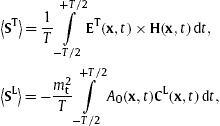to find
equation3.7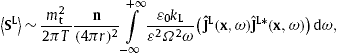from which the transversal and longitudinal Cherenkov flux densities can be extracted, cf. Section 4.

## 4. Superluminal radiation by an inertial charge in a dispersive spacetime

We consider a classical point charge q in uniform motion x0(t)=υt, with subluminal speed υ<1. The charge and current densities are ρ=qδ(xυt) and j(x,t)=qυδ(xυt). We use the Heaviside–Lorentz system, so that αe=e2/(4πc)≈1/137 and αq=q2/(4πc) are the electric and tachyonic fine-structure constants. The current transform (3.3) reads

where the time cutoff T→∞ has been introduced as a regularization. The time integration is performed by means of the limit definition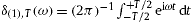of the Dirac function. A second limit definition, , is invoked in (4.2) to calculate the time-averaged flux vectors. We writeand use υ as polar axis, , to find, cf. (3.7),
equation4.2Performing the limit T→∞, we can replace δ(2),T by the ordinary delta function.

The radiant transversal/longitudinal power is obtained by integrating the Poynting vectors (4.2) over a sphere of radius r→∞, . By interchanging the dθ and dω integrations, we find

where pT,L(ω) are the tachyonic Cherenkov densities for transversal/longitudinal radiation, The integration range in (4.3) is actually over positive ω intervals in which ω/(kT,L(ω)υ)⩽1, cf. (3.2). From now on, we will use constant (i.e. frequency-independent) permeabilities (ε,μ) and (ε0,μ0) as well as a constant tachyonic mass-square . In this case, the integration range in (4.3) is defined by cutoff frequencies ωT,L,max obtained as the solutions of kT,L(ω)υ=ω, respectively.

In the tachyonic spectral densities (4.4), we substitute the wave numbers (3.2), and parametrize the particle velocity with the Lorentz factor, :

where we use the shortcut
equation4.6We also define ηL(γ) analogous to ηT(γ) with the product εμ in (4.6) replaced by ε0μ0. In the following, we restrict to permeabilities satisfying εμ⩽1 and ε0μ0⩽1, since the spectral averaging carried out in the next section leads to exponentially decaying spectral densities only in this case. Subject to these constraints, the functions ηT,L(γ) are positive, irrespectively of the Lorentz factor γ⩾1, and related to the cutoff frequencies in (4.3) by .

## 5. Tachyonic Cherenkov densities averaged with relativistic electron distributions

We rescale the frequency in the radiation densities (4.5), ,

equation5.1where we have introduced the frequency-dependent tachyonic fine-structure constant αq(ω)=q2/(4πΩ2(ω)). The scale factor Ω2(ω), cf. after (2.5), is chosen as
equation5.2where the exponent σ is to be determined from an empirical spectral fit. We note Ω(ω)→1 for ω→∞ as well as mt→0, and . The permeabilities ε,μ,ε0,μ0 are positive and constant, satisfying εμ⩽1 and ε0μ0⩽1, cf. after (4.6). The transversal/longitudinal spectral range is 0⩽ωωT,L,max(γ), with . The cutoff factors ηT,L(γ) in (4.6) are monotonically increasing, from zero at γ=1 to the maximum ηT(∞)=[εμ0(1/(εμ)−1)]−1 and ηL(∞)=[εμ0(1/(ε0μ0)−1)]−1, respectively.

We average the radiation densities (5.1) with an electronic power-law distribution , ,  and ,

equation5.3where the dimensionless normalization constant Aα,β is related to the electronic number count Ne by The exponential cutoff β=me/(kBT) determines the electron temperature, T[K]≈5.93×109/β. kB is the Boltzmann constant and me the electron mass. A thermal Maxwell–Boltzmann distribution requires the electron index α=−2.

The spectral average of the transversal radiation densities in (5.1) is carried out as

equation5.5
where θ is the Heaviside step function. The same applies to the longitudinal density, if we replace T→L and εμε0μ0. To evaluate integral (5.5), we solve the inequality ω<ωT,max, , for γ, cf. (4.6): which is valid if the denominator is positive. As pointed out after (5.2), only frequencies in the range 0<ω<ωT,max(∞) can be radiated, where . In this frequency range, the denominator in (5.6) is positive and γT,min(ω) is monotonically increasing, reaching infinity at ωT,max(∞). Thus, for a frequency in the range 0<ω<ωT,max(∞) to be radiated, this requires the electronic Lorentz factor γ to exceed γT,min(ω). This holds true for longitudinal radiation as well, if we perform the substitutions T→L and εμε0μ0, which also define γL,min(ω) via (5.6).

With these prerequisites, the average (5.5) can be reduced to the spectral functions 

so that, cf. (5.6),
equation5.8
pT(ω)〉α,β=θ(ωT,max(∞)−ω)BT(ω,γT,min(ω)).In the Heaviside function θ, it is convenient to rescale the argument, writing , where . The longitudinal average pL(ω)〉α,β is obtained by the substitutions T→L and εμε0μ0 in (5.6) and (5.8).

The spectral functions BT,L(ω,γ1) in (5.7) admit integration in terms of incomplete gamma functions,

equation5.9where αq(ω) is the tachyonic fine-structure constant defined before (5.2). The longitudinal spectral function reads
equation5.10We note that Γ is elementary for Maxwell–Boltzmann averages, α=−2, Γ(1,βγ1)=eβγ1, and it decays exponentially for βγ1≫1, Γ(−α−1,βγ1)∼(βγ1)α−2eβγ1. Accordingly, BT(ω,γT,min(ω)) decays exponentially as well, since γT,min(ω) diverges for ωωT,max(∞), cf. (5.6), and the same holds true for BL(ω,γL,min(ω)) and ωωL,max(∞). In the low-frequency limit, , we find , cf. (5.2).

## 6. Tachyonic spectral fit to Jupiterʼs radio emission

### 6.1. Superluminal Cherenkov flux in the radio band

We restore the units =c=1 and use eV units for the tachyon mass, so that mt stands for mtc2[eV]. As for the radiated frequencies, we put , where . The energy-dependent tachyonic fine-structure constant is dimensionless, αq(ω)=αt/Ω2(ω), cf. (5.2); the proportionality factor αt=q2/(4πc) is the tachyonic counterpart to the electric fine-structure constant e2/(4πc), αq(ω→∞)=αt. The permeabilities (2.1) and the temperature parameter β are dimensionless. The spectral functions BT,L in (5.9) and (5.10) and the averaged densities pT,L(ω)〉α,β in (5.8) are in eV units accordingly. The power transversally and longitudinally radiated is thus , where we substitute mtmtc2[eV] and in the integrand. The transversal/longitudinal flux densities read

equation6.1where d[cm] is the distance to the radiating source. The total unpolarized flux density is . Jupiterʼs standard geocentric distance is d≈4.04 AU≈6.04×1013 cm ,  and . As we have already expressed pT,L(ω)〉α,β in terms of the rescaled frequency , we only need to substitute in the spectral densities (5.8), where ν is measured in hertz, and κt,Hz[s] is a fitting parameter, , determining the tachyon mass in the radio band.

We note the conversions ν[GHz]≈2.418×105E[eV] and 1 Jy≈6.2415×10−12 eV/(cm2), so that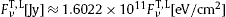. To better distinguish linear spectral slopes from curved spectral cutoffs, one can use the rescaled flux density , where k is preferably a positive integer exponent. In Fig. 1, we plot against ν[GHz], and the fit is performed with the total flux density .Fig. 1.

Tachyonic Cherenkov fit to the Jovian radio emission. Data points of the 1998 multi-site campaign from , VLA (Very Large Array), DSN (Deep Space Network) and Cassini 2001 data from . The fit T + L (solid curve) depicts the total tachyonic flux density rescaled with frequency. This unpolarized density is obtained by adding the transversal flux component (dotted curve labeled T) and the longitudinal component (dashed curve L), generated by a thermal electron plasma, cf. (6.2). Jupiterʼs radio spectrum shows an extended power-law ascent terminating in a spectral peak around 6 GHz, which is followed by an exponentially decaying tail inferred from the Cassini data point at 13.8 GHz. The fitting parameters are recorded in Section 6.2.

Summarizing the flux densities employed in the spectral fit in Fig. 1, we assemble in the above stated units. The transversal Cherenkov density (6.1) reads

equation6.2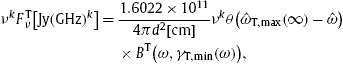where , cf. after (5.8). The spectral function BT in (5.9) and the argument γT,min(ω) in (5.6) are already expressed in the rescaled variable . The longitudinal counterpart to (6.2) is obtained by replacing T→L and εμε0μ0, so that .

In (6.2), we substitute , where the frequency ν is measured in GHz so that κt=109κt,Hz or, cf. after (6.1),

In this parametrization, we find the transversal spectral function (5.9) as
equation6.4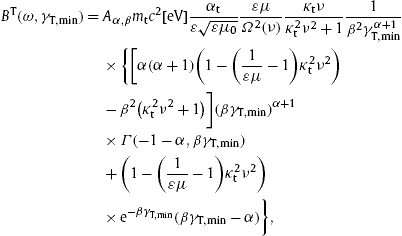and the longitudinal spectral function in (5.10) reads
equation6.5In (6.4) and (6.5), we have to insert the frequency-dependent minimal Lorentz factors, cf. (5.6),
equation6.6and the scale factor
equation6.7of the tachyonic fine-structure constant αq(ω)=αt/Ω2, αt=q2/(4πc), see (5.2) and before (6.1).

The Heaviside function in the flux density (6.2) can be replaced by . The highest frequency transversally/longitudinally radiated is νT,max=(1/(εμ)−1)−1/2/κt and νL,max=(1/(ε0μ0)−1)−1/2/κt respectively, cf. after (6.2). The tachyonic mass parameter κt is defined in (6.3). The constant amplitudes in (6.2) and (6.4) can be combined to one fitting parameter,

with 4πd2≈4.58×1028 cm2 for Jupiter, cf. after (6.1). This amplitude at can also be used for the longitudinal radiation component in (6.2) and (6.5).

### 6.2. Spectral asymptotics and tachyonic Cherenkov fit of the Jovian radio emission

We consider a thermal electron distribution (5.3) with electron index α=−2, and permeabilities (2.1) satisfying εμ=ε0μ0=1. (This is slightly more general than vacuum permeabilities ε=μ=1, ε0=μ0=1.) The transversal spectral function BT(ω,γT,min) in (6.4) then simplifies to

equation6.9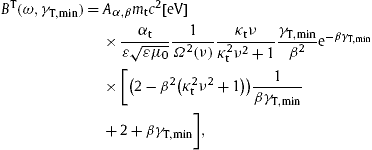and its longitudinal counterpart in (6.5) is
equation6.10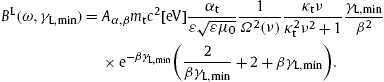The minimal Lorentz factor to be substituted is , cf. (6.6), and the fine-structure scale factor Ω2(ν) is stated in (6.7). ν is measured in GHz, and the tachyonic mass parameter κt is defined in (6.3).

The flux densities in (6.2) apply, with the Heaviside function dropped. In the low-frequency limit κtν→0, we find the unpolarized flux

The parameter at is defined in (6.8). We can estimate the amplitude A0 and the exponent σ by fitting this power-law slope, which is linear in a log–log plot, to the low-frequency spectrum. In the asymptotic high-frequency limit κtν≫1, the unpolarized flux reads
equation6.12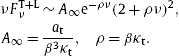An initial estimate of A and ρ is obtained by fitting this exponentially decaying flux in the high-frequency regime. Once the parameters A0,∞, σ and ρ have been estimated from the asymptotic spectral fits, we find the temperature parameter β of the radiating electron population by solving
equation6.13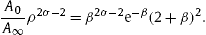This equation readily follows from the definition of the asymptotic fitting parameters A0,∞ and ρ in (6.11) and (6.12). Initial values for κt and at are found as κt=ρ/β and at=Aβ2ρ.

The least-squares fit of the flux densities (6.2) is performed by varying the parameters A0,∞,ρ and σ in the vicinity of their initial values obtained from the asymptotic fits (6.11) and (6.12); the corresponding β is obtained by solving (6.13). In addition, we may vary the permeabilities in the vicinity of εμ=ε0μ0=1, subject to the constraints εμ⩽1 and ε0μ0⩽1, cf. after (4.6). The electron index can also be varied around its equilibrium value α=−2, cf. (5.3), by employing the nonthermal spectral functions (6.4) and (6.5) instead of (6.9) and (6.10), but this is not necessary for the Jovian radio spectrum.

The tachyonic Cherenkov fit of Jupiterʼs radio emission depicted in Fig. 1 is performed with a thermal electron distribution α=−2 and permeabilities εμ=ε0μ0=1. The numerical values of the fitting parameters are

equation6.14The fine-structure scaling exponent σ is defined in (6.7), the temperature parameter β in (5.3), the tachyonic mass parameter κt[s] in (6.3), and the flux amplitude at[eV/cm2] in (6.8). In practice, we use the parameters of the asymptotic flux limits (6.11) and (6.12) as fitting parameters, which are A0≈4.9, A≈4.0×109, σ≈0.5 and ρ≈0.8. The parameters in (6.14) have been calculated from these values as explained above.

The electron temperature is T[K]≈2.51×108, cf. after (5.4), which is the only point where the electron mass enters, via β=me/(kBT). (The mass of the radiating particle does not show in the classical Cherenkov densities (4.5), in contrast to the tachyon mass mt.) Assuming vacuum permeabilities, εμ≈1, ε0μ0≈1, we can estimate the tachyon mass in the radio band, mtc2[eV]≈1.22×10−4 or mtc2≈29.5 GHz, cf. (6.3) and (6.14). From the amplitude at in (6.8) and the Jovian distance estimate, we find the product of the asymptotic tachyonic fine-structure constant αt (defined before (6.1)) and the normalization factor of the electron distribution (5.3) as Aα,βαt≈4.17×1033. The integral Kα,β in (5.4) determining the electron number Ne=Aα,βKα,β is calculated with the temperature parameter β in (6.14), Kα=−2,β≈6.65×10−13. The estimate for the product of electron count and tachyonic fine-structure constant is thus Neαt≈2.77×1021.

## 7. Conclusion

We have investigated the emission of tachyonic radiation modes by freely propagating electrons in a dispersive spacetime. The superluminal group velocity υT,L=dω/dkT,L(ω) is caused by the negative mass-square in the wave numbers (3.2) of the tachyonic radiation field. υT,L differs for transversal and longitudinal modes , unless the wave numbers coincide, which requires permeabilities satisfying ε0μ0=εμ. The tachyonic wavelength is λT,L=2π/kT,L. In the radio band and with vacuum permeabilities, we find the group velocity and wavelength , with ν in GHz. A tachyon mass mt of 29.5 GHz is inferred from the spectral fit in Fig. 1, cf. after (6.14).

We introduced tachyonic field strengths and inductions defined by constitutive relations with frequency-dependent permeabilities. We then developed an equivalent 4D space–frequency representation of the dispersive radiation field, deriving manifestly covariant field equations. Thus the suggestive and efficient formalism of manifest covariance can be maintained, but the underlying space conception is non-relativistic, as superluminal wave propagation requires an absolute spacetime conception to preserve causality ,  and .

We focused on superluminal Cherenkov radiation, the tachyonic radiation field being generated by a classical subluminal charge uniformly moving in a permeable spacetime. The electron mass does not enter in the classical radiation densities (4.4), which suggests that the tachyonic quanta are actually emitted by the medium stimulated by the field of the moving electron  and . A longitudinal radiation component emerges, with amplitude proportional to the negative mass-square of the tachyonic modes. We parametrized the Cherenkov flux densities with the Lorentz factor of the inertial charge, averaged them with a relativistic electron distribution, and explained how to perform tachyonic spectral fits in the radio band.

A flux average over a mildly relativistic thermal electron population (with Maxwell–Boltzmann distribution) suffices to model the currently observed Jovian radio spectrum. We also calculated the tachyonic Cherenkov flux radiated by nonthermal electron populations (with power-law distribution), cf. Section 5, which can be useful to model X- and γ-ray spectra ,  and . As for Jupiter, the low flux density at 13.8 GHz (Cassini 2001 in-situ measurement  and ) is caused by an exponential spectral cutoff. The low-frequency spectrum is linear in the double-logarithmic flux representation of Fig. 1. The cross-over region around the spectral peak at 6 GHz is also well reproduced by the tachyonic flux densities (4.4) averaged over a thermal electron population.

## References

• 
• Electrodynamics of Continuous Media

• Pergamon, Oxford (1984)

• 
• Transition Radiation and Transition Scattering

• Hilger, Bristol (1990)

• 
• Sov. Phys. Usp., 15 (1972), p. 184